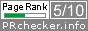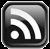# LEARN MS-ACCESS TIPS AND TRICKS

Learn Microsoft Access Advanced Programming Techniques, Tips and Tricks.

### Introduction

The other day one of my colleagues asked me to open MS-Word and type the expression =Rand() on a separate line and press the Enter key. It was magic that I didn't know till that time. The following sentence appears fifteen times repeatedly (in 3 rows and 5 columns) overwriting the expression itself:

The quick brown fox jumps over the lazy dog.

Open a Document in MS-Word and try it out yourself. The above sentence has all the letters of the alphabet in it. You can control the printing by inputting parameters to the Function like =Rand(5,1) will print the same sentence in 5 lines in one Column. It is a built-in Function with different constructs that can accept a different set of parameters and looks like created for fun, I think! It works only when you type it on a separate line. Even though it looks like a Random Function it has nothing to do with it.

There is another feature in MS-Word that, I like to see in MS-Access, formats numeric values in Cardinal Text Format.

For example, the result of the Mail Merge formula { = 9.20 + 5.35 \* CardText } outputs fourteen and 55/100, when the document is merged into another Document or to Printer. Format switches can be either \* DollarText or \* CardText to get the above output. When the \* Caps switch is added to it, like { = 9.20 + 5.35 \* DollarText \* Caps} , then it changes the first letter of each word into upper case.

This is very useful for printing Invoice values in MS-Access. I have written a Function to achieve this in MS-Access and here it is for you to try it out.

### The CardText() Function

Copy and Paste the following Code into a Global Module of your Database and save it:

```Public Function CardText(ByVal inNumber As Double, Optional ByVal precision As Integer = 2) As String
'------------------------------------------------------------------------
'Author : a.p.r. pillai
'Date   : December 2008
'URL    : www.msaccesstips.com
'------------------------------------------------------------------------
Dim ctu, ctt, bmth
Dim strNum As String, j As Integer, k As Integer, fmt As String
Dim h As Integer, xten As Integer, yten As Integer
Dim cardseg(1 To 4) As String, txt As String, d As String, txt2 As String
Dim locn As Integer, xfract As String, xhundred As String
Dim xctu As String, xctt As String, xbmth As String

On Error GoTo CardText_Err

strNum = Trim(Str(inNumber))
locn = InStr(1, strNum, ".")
'Check Decimal Places and rounding
If locn > 0 Then
xfract = Mid(strNum, locn + 1)
strNum = Left(strNum, locn - 1)
If precision > 0 Then
If Len(xfract) < precision Then
xfract = xfract & String((precision - Len(xfract)), chr(48))
ElseIf Len(xfract) > precision Then
xfract = Format(Int(Val(Left(xfract, precision + 1)) / 10 + 0.5), String(precision, "0"))
End If
xfract = IIf(Val(xfract) > 0, xfract & "/" & 10 ^ precision, "")
Else
strNum = Val(strNum) + Int(Val("." & xfract) + 0.5)
xfract = ""
End If
End If

h = Len(strNum)
If h > 12 Then
'if more than 12 digits take only 12 (max. 999 Billion)
'extra value will get truncated from left.
strNum = Right(strNum, 12)
Else
strNum = String(12 - h, "0") & strNum
End If

GoSub initSection

txt2 = ""
For j = 1 To 4
If Val(cardseg(j)) = 0 Then
GoTo NextStep
End If
txt = ""
For k = 3 To 1 Step -1
Select Case k
Case 3
xten = Val(Mid(cardseg(j), k - 1, 1))
If xten = 1 Then
txt = ctu(10 + Val(Mid(cardseg(j), k, 1)))
Else
txt = ctt(xten) & ctu(Val(Mid(cardseg(j), k, 1)))
End If
Case 1
yten = Val(Mid(cardseg(j), k, 1))
xhundred = ctu(yten) & IIf(yten > 0, bmth(1), "") & txt
Select Case j
Case 2
d = bmth(2)
Case 3
d = bmth(3)
Case 4
d = bmth(4)
End Select
txt2 = xhundred & d & txt2
End Select
Next
NextStep:
Next

If Len(txt2) = 0 And Len(xfract) > 0 Then
txt2 = xfract & " only. "
ElseIf Len(txt2) = 0 And Len(xfract) = 0 Then
txt2 = ""
Else
txt2 = txt2 & IIf(Len(xfract) > 0, " and " & xfract, "") & " only."
End If

CardText = txt2

CardText_Exit:
Exit Function

initSection:
xctu = ", One, Two, Three, Four, Five, Six, Seven, Eight, Nine, Ten, Eleven, Twelve,"
xctu = xctu & " Thirteen, Fourteen, Fifteen, Sixteen, Seventeen, Eighteen, Nineteen"
ctu = Split(xctu, ",")

xctt = ", Ten, Twenty, Thirty, Fourty, Fifty, Sixty, Seventy, Eighty, Ninety"
ctt = Split(xctt, ",")

xbmth = ", Hundred, Thousand, Million, Billion"
bmth = Split(xbmth, ",")
k = 4
For j = 1 To 10 Step 3
cardseg(k) = Mid(strNum, j, 3)
k = k - 1
Next
Return

CardText_Err:
CardText = ""
MsgBox Err.Description, , "CardText()"
Resume CardText_Exit
End Function
```

CardText or DollarText

### Sample Demo Runs

The Function name CardText() is derived from MS-Word Number Format Switch \* CardText. The CardText() Function can accept a maximum value of 10^12-1 or up to 999 Billion. For most applications, this will be sufficient. Passing a Value greater than this will get truncated from left.

The CardText() Function accepts two parameters and the second one is Optional. The second parameter controls the number of digits after decimal places.

By default, the CardText() Function will round-off fractional part, if present, to two decimal places when the second parameter is omitted.

To try out the Code you may open VBA Editing Window (Alt+F11) and open Immediate Window (Ctrl+G) and type the following statement or similar one with a different Value or Expression:

Example:? CardText(1234.5678,3) will produce the result shown below.

Result: One Thousand Two Hundred Thirty-Four and 568/1000 only.

The first parameter can be a Number or an Expression that evaluates a Numeric Value. If the second parameter is zero then the Number is rounded to the next highest Integer.

Example: ? CardText(1234.5678,0)

Result: Thousand Two Hundred Thirty-Five only.

To change the output to upper-case or lower-cases letters enclose the CardText() Function in UCase() or LCase() built-in function respectively.

Example: ? UCase(CardText(1234.5678))

Result: ONE THOUSAND TWO HUNDRED THIRTY-FOUR AND 57/100 ONLY.

To prefix a Currency Description use the following example:

Example: ? "Dollars" & CardText(1234.5678)

Or

="Dollars" & CardText([UnitPrice]) on Forms  or  Reports.

Result: Dollars One Thousand Two Hundred Thirty-Four and 57/100 only.

You may try the Function on Form or Report with data field Value as input.

The CardText() Function is not extensively field-tested and if you find bugs please let me know. Use it at your own risk.

Any suggestions for improvement are welcome.

Share:

Comments subject to moderation before publishing.

## TranslateSubscribe in a readerYour email address:

Delivered by FeedBurner